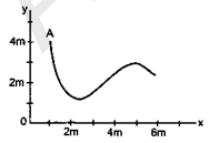# A particle starts from a point A and travels along`
Question:

A particle starts from a point $A$ and travels along the solid curve shown in the given figure. Find approximately the position $\mathrm{B}$ of the particle such that the average velocity between the positions $\mathrm{A}$ and $\mathrm{B}$ has the same direction as the instantaneous velocity at $\mathrm{B}$.Solution:

Direction of instantaneous velocity of point $\mathrm{B}$ must be same as direction of average velocity $\overrightarrow{\mathrm{AB}}$. So, point is approximately $(5,3)$